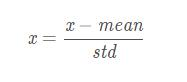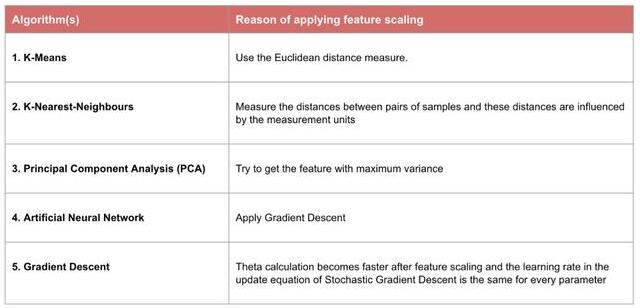# Python3 常用數據標準化方法詳解## 一、[0, 1] 標準化

[0, 1] 標準化是最基本的一種數據標準化方法，指的是將數據壓縮到0～1之間。```def MaxMinNormalization(x, min, max):
"""[0,1] normaliaztion"""
x = (x - min) / (max - min)
return x```

```def MaxMinNormalization(x):
"""[0,1] normaliaztion"""
x = (x - np.min(x)) / (np.max(x) - np.min(x))
return x```

## 二、Z-score標準化

Z-score標準化是基於數據均值和方差的標準化化方法。標準化後的數據是均值為0，方差為1的正態分佈。這種方法要求原始數據的分佈可以近似為高斯分佈，否則效果會很差。```def ZscoreNormalization(x, mean_, std_):
"""Z-score normaliaztion"""
x = (x - mean_) / std_
return x```

```def ZscoreNormalization(x):
"""Z-score normaliaztion"""
x = (x - np.mean(x)) / np.std(x)
return x```

## 數據預處理

### 常用的方法有兩種：Z-Score標準化：將原始數據映射到均值為0、標準差為1的分佈上## 為什麼要標準化/歸一化？### 哪些機器學習算法需要標準化和歸一化

1）需要使用梯度下降和計算距離的模型要做歸一化，因為不做歸一化會使收斂的路徑程z字型下降，導致收斂路徑太慢，而且不容易找到最優解，歸一化之後加快瞭梯度下降求最優解的速度，並有可能提高精度。比如說線性回歸、邏輯回歸、adaboost、xgboost、GBDT、SVM、NeuralNetwork等。需要計算距離的模型需要做歸一化，比如說KNN、KMeans等。

2）概率模型、樹形結構模型不需要歸一化，因為它們不關心變量的值，而是關心變量的分佈和變量之間的條件概率，如決策樹、隨機森林。### 徹底理解標準化和歸一化```#導入數據
import numpy as np
import matplotlib.pyplot as plt
import pandas as pd
df = pd.read_csv('Data.csv')```

### 缺失值均值填充,處理字符型變量

```df['Salary'].fillna((df['Salary'].mean()), inplace= True)
df['Age'].fillna((df['Age'].mean()), inplace= True)
df['Purchased'] = df['Purchased'].apply(lambda x: 0 if x=='No' else 1)
df=pd.get_dummies(data=df, columns=['Country'])```### 最大 – 最小規范化

```from sklearn.preprocessing import MinMaxScaler
scaler = MinMaxScaler()
scaler.fit(df)
scaled_features = scaler.transform(df)
df_MinMax = pd.DataFrame(data=scaled_features, columns=["Age", "Salary","Purchased","Country_France","Country_Germany", "Country_spain"])```### Z-Score標準化

```from sklearn.preprocessing import StandardScaler
sc_X = StandardScaler()
sc_X = sc_X.fit_transform(df)
sc_X = pd.DataFrame(data=sc_X, columns=["Age", "Salary","Purchased","Country_France","Country_Germany", "Country_spain"])``````import seaborn as sns
import matplotlib.pyplot as plt
import statistics
plt.rcParams['font.sans-serif'] = ['Microsoft YaHei']
fig,axes=plt.subplots(2,3,figsize=(18,12))
sns.distplot(df['Age'], ax=axes[0, 0])
sns.distplot(df_MinMax['Age'], ax=axes[0, 1])
axes[0, 1].set_title('歸一化方差：% s '% (statistics.stdev(df_MinMax['Age'])))
sns.distplot(sc_X['Age'], ax=axes[0, 2])
axes[0, 2].set_title('標準化方差：% s '% (statistics.stdev(sc_X['Age'])))
sns.distplot(df['Salary'], ax=axes[1, 0])
sns.distplot(df_MinMax['Salary'], ax=axes[1, 1])
axes[1, 1].set_title('MinMax：Salary')
axes[1, 1].set_title('歸一化方差：% s '% (statistics.stdev(df_MinMax['Salary'])))
sns.distplot(sc_X['Salary'], ax=axes[1, 2])
axes[1, 2].set_title('StandardScaler:Salary')
axes[1, 2].set_title('標準化方差：% s '% (statistics.stdev(sc_X['Salary'])))```